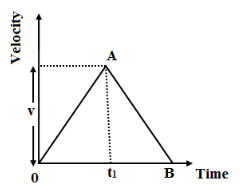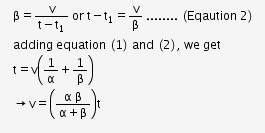# A car accelerates from rest at a constant rate α for some time

A car accelerates from rest at a constant rate α for some time after which it decelerates at a constant rate β and comes to rest. if the total time elapsed is ‘t’ then prove that maximum velocity acquired by the car isFrom rest, the car accelerates for a timr t1.

Thus, its velocity-time graph is a staright line OA sloping in the upward direction.the maximum velocity of the car be considered to be ‘v’.

The slope of the graph OA gives the accelration of the car.The car begins decelerate after attaining the maximum velocity.Thus, its velocity-time graph is a straight line AB sloping in th edownward direction.The slope of the graph AB gives the detardation of the car.